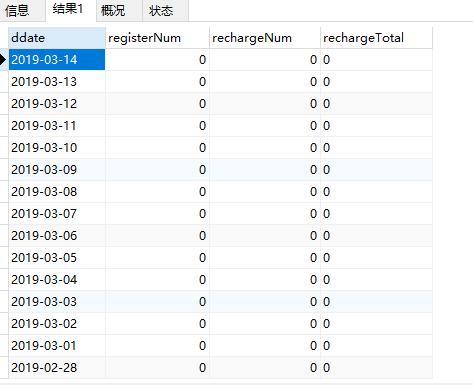CREATE TABLE num (i int);
INSERT INTO num (i) VALUES (0), (1), (2), (3), (4), (5), (6), (7), (8), (9);
CREATE TABLE  if not exists calendar(datelist date);  INSERT INTO calendar(datelist) SELECT
(
DATE_FORMAT("2019-1-1", '%Y-%m-%d')
),
numlist.id
) AS date
FROM
(
SELECT
n1.i + n10.i * 10 + n100.i * 100 + n1000.i * 1000+ n10000.i * 10000 AS id
FROM
num n1
CROSS JOIN num AS n10
CROSS JOIN num AS n100
CROSS JOIN num AS n1000
CROSS JOIN num AS n10000
) AS numlist;

SELECT
date(dday) ddate,
max(registerNum) as registerNum,
max(rechargeNum) as rechargeNum,
max(rechargeTotal) as rechargeTotal
FROM
(
SELECT
datelist as dday,0 as registerNum,0 as rechargeNum,0 as rechargeTotal
FROM
calendar
WHERE  1  AND DATE_SUB(CURDATE(), INTERVAL 365 DAY) <= date(datelist)&&date(datelist)<=CURDATE()
UNION ALL
SELECT  FROM_UNIXTIME(a.time,"%Y-%m-%d") as dday, 0 as registerNum,count(DISTINCT(a.user_id)) as rechargeNum,sum(a.money) as rechargeTotal FROM
top_up AS a LEFT  JOIN referee AS b ON a.user_id=b.referee_id
LEFT JOIN channel_user AS c ON b.user_id = c.uid WHERE 1  AND c.uid=1087 AND a.status=2
GROUP BY dday
UNION ALL
SELECT  FROM_UNIXTIME(a.time,"%Y-%m-%d") as dday, count(a.referee_id) as registerNum,0 as rechargeNum,0 as rechargeTotal FROM
referee AS a
LEFT JOIN channel_user AS b ON a.user_id = b.uid WHERE 1  AND b.uid=1087
GROUP BY dday
) a
GROUP BY ddate
ORDER BY ddate DESC LIMIT 0,10